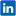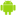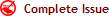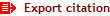|Twitter |Facebook |Google+ |VKontakte | LinkedIn|Viadeo | English | Français | Español | العربية |International Journal of Innovation and Applied Studies
ISSN: 2028-9324     CODEN: IJIABO     OCLC Number: 828807274     ZDB-ID: 2703985-7
 Saturday 08 August 2020

# Connect with IJIAS

••••••••Now IJIAS is indexed in EBSCO, ResearchGate, ProQuest, Chemical Abstracts Service, Index Copernicus, IET Inspec Direct, Ulrichs Web, Google Scholar, CAS Abstracts, J-Gate, UDL Library, CiteSeerX, WorldCat, Scirus, Research Bible and getCited, etc.

# RESOLUTION OF SYSTEMS OF LINEAR DIFFERENTIAL EQUATIONS ON CONSTANT COEFFICIENTS

[ RESOLUTION DES SYSTEMES D’EQUATIONS DIFFERENTIELLES LINAIRES A COEFFICIENTS CONSTANTS ]

Volume 18, Issue 1, October 2016, Pages 191–214Théodore Mapendo Wendo1

1 Département de Mathématique-physique, Institut Supérieur Pédagogique d’Idjwi (ISP-IDJWI), Idjwi, Sud-Kivu, RD Congo

Original language: French

Copyright © 2016 ISSR Journals. This is an open access article distributed under the Creative Commons Attribution License, which permits unrestricted use, distribution, and reproduction in any medium, provided the original work is properly cited.

# Abstract

In mathematical analysis, methods were developed for solving differential equations as there is no general method for solving these equations. However, in Algebra, the simultaneous equations are those for which the roots all check equations, they form the system of equations. We note in the study of differential equations that the system of differential equations is not very developed yet many books to refer analysis but not explicitly. And these systems can be put in matrix form. This allows the resolution of these systems by using the matrices being given various operations on the matrix and determinant calculation. The ideal of this article is to make the public interested in mathematics, clear text with a more understandable language on solving linear ordinary differential equations systems with constant coefficients. Our research is limited to the case of linear differential equations with constant coefficients. We do not claim to theorize on solving ordinary linear differential equation systems with constant coefficients less and extend applications.

Author Keywords: homogeneous equation, inhomogeneous equation, differential system, solving a normal system.

# Abstract: (french)

En Analyse mathématique, des méthodes sont développées pour la résolution des équations différentielles car il n’existe pas de méthode générale de résolution pour toutes ces équations. Cependant, en Algèbre, les équations simultanées sont celles pour lesquelles les racines vérifient toutes les équations, elles forment le système d’équations. Nous remarquons pendant l’étude des équations différentielles que, le système d’équations différentielles n’est pas très développé pourtant beaucoup de livres d’Analyse en font référence mais de manière non explicite. Et ces systèmes peuvent se mettre sous forme matricielle. Ce qui permet la résolution de ces systèmes en se servant des matrices étant données diverses opérations sur le calcul matriciel et déterminant. L’idéal de cet article est de mettre à la disposition du public intéressé par les mathématiques, un texte clair avec un langage plus compréhensible sur la résolution des systèmes d’équations différentielles linéaires ordinaires à coefficients constants. Notre recherche se limite au cas de système d’équations différentielles linéaires à coefficients constants. Nous n’avons pas de prétention à élaborer une théorie sur la résolution les systèmes d’équation différentielles linéaires ordinaires à coefficients constants moins en étendre des applications.

Author Keywords: équation homogène, équation non homogène, système différentiel, résolution d’un système normal.